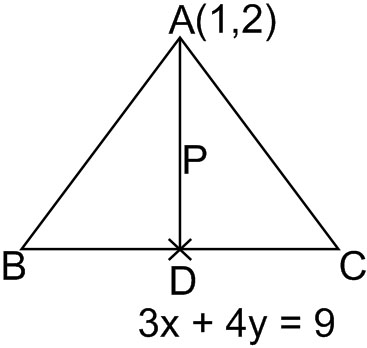#### The base of an equilateral triangle is along the line given by 3x + 4y = 9. If a vertex of the triangle is (1, 2), then the length of a side of the triangle is : Option 1)Option 2)Option 3)Option 4)As we learnt in

Perpendicular distance of a point from a line -- whereinis the distance from the line.AlsoThusOption 1)This option is incorrect.

Option 2)This option is correct.

Option 3)This option is incorrect.

Option 4)This option is incorrect.

#### divya.saini